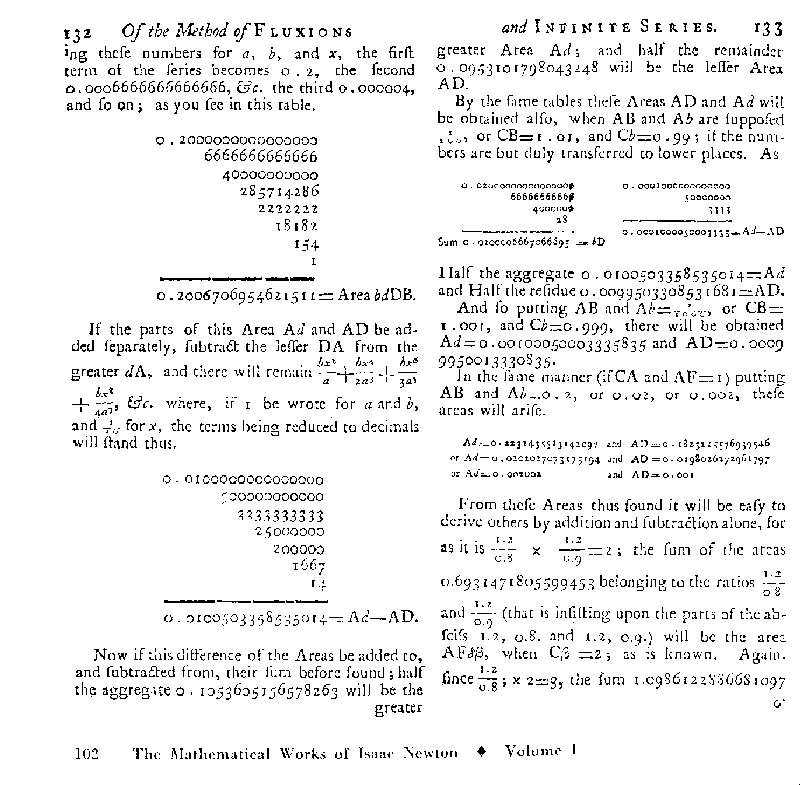# Notes on Newton's estimates for the Hyperbolic Logarithm.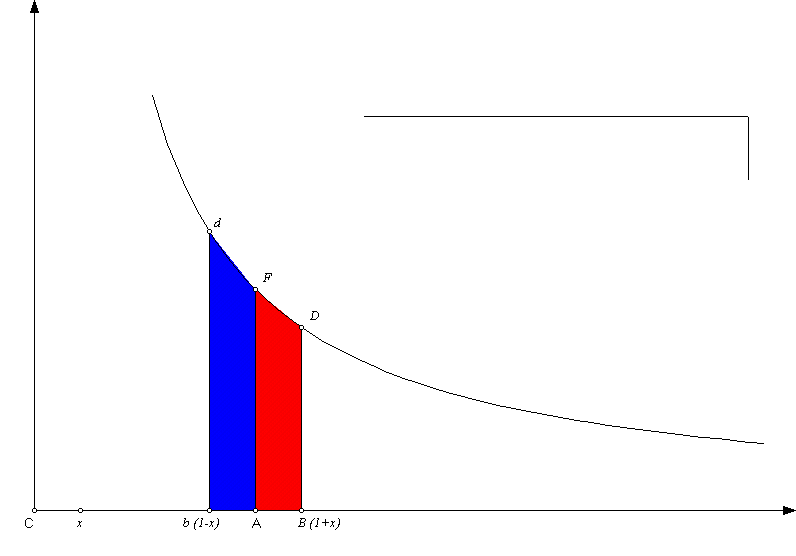Newton considers symmetrically located points on the main axis, 1+x and 1-x with x>0 and their related reciprocals.
He then uses two integrals related to the geometric series to determine the related areas,
(i) between the hyperbola and above the segment [1,1+x] (red) and
(ii) between the hyperbola and above the segment [1-x, 1] (blue):

(i)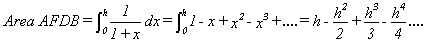(ii)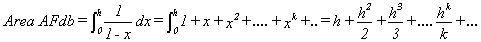These allow the estimation of the sum and difference of the two areas: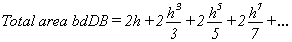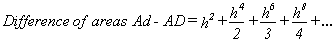.

Now Newton uses the first eight terms with h = .1 (and .2) to estimate the hyperbolic log of .9, 1.1 (.8 , and 1.2).

[Tables created with Microsoft Excel]

 h 0.1 0.2 .01 2h 0.2 0.4 0.02 2h3/3 0.000666667 0.00533333 0.00000066666666667 2h5/5 4e-06 0.000128 0.00000000004 2h7/7 2.85714e-08 3.65714e-06 0.00000000000000286 2h9/9 2.22222e-10 1.13778e-07 2.22222222222e-19 2h11/11 1.8182e-12 3.72364e-09 2h13/13 1.54e-14 1.26031e-10 2h15/15 1e-16 4.36907e-12 Sum of Areas 0.200671 0.405465 0.020000666706670
 h 0.1 0.2 .01 h2 0.01 0.04 0.0001 h4/2 5e-05 0.0008 0.000000005 h6/3 3.33333e-07 2.13333e-05 0.000000000000333 h8/4 2.5e-09 6.4e-07 2.50000000000e-17 h10/5 2e-11 2.048e-08 h12/6 1.667e-13 6.8267e-10 h14/7 1.4e-15 2.341e-11 Diff'ce of Areas 0.0100503 0.040822 0.000100005000333
Now to find the Area of the two separate regions (and related logarithms) we take 1/2 of the difference of these results and 1/2 of the sum of these results.

Thus ln(1.1)= 1/2 ( 0.2006706954621511- 0.0100503358535014)

= 0.0953101798043248

while ln(.9) = -(1/2)( 0.2006706954621511 + 0.0100503358535014)

= -0.105360516578263 .

And ln(1.2)= 1/2 (0.405465108108002 -0.040821994519406 )

= 0.18232155576939546 (from Newton)

while ln(.8) = -(1/2)(0.405465108108002 +0.040821994519406 )

= -0.2231435513142097 (from Newton) .

Newton can also find ln(1.01) and ln(.99) by using h=.01 from the same tables.

Then Newton finds other logarithms with great accuracy using these.

For Example: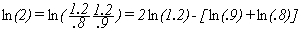= (approx) 2(0.18232155576939546) +0.105360516578263+ 0.2231435513142097

= 0.6931471805599453 (from Newton)